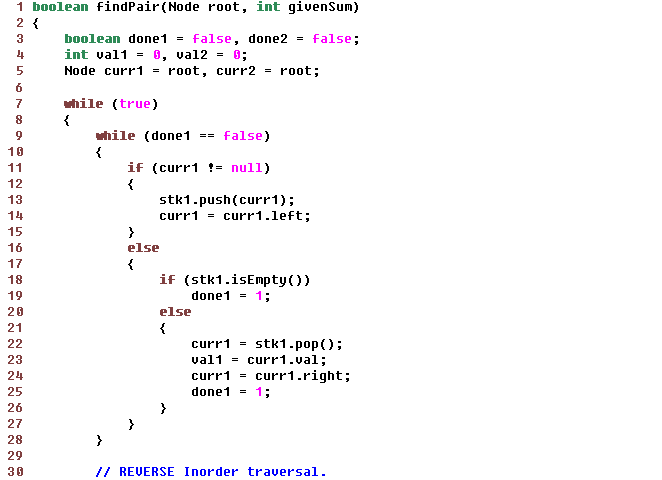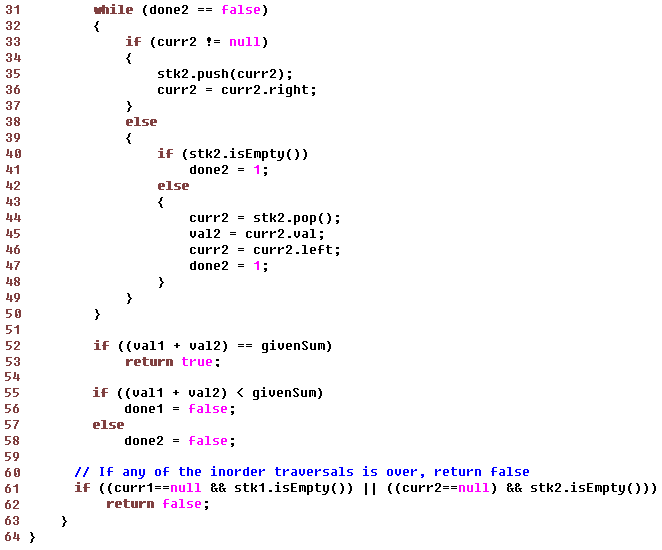Data Structuresand Algorithms Good OldJava AdvancedInterview Topics Cloud andDatabases Web Designand Development Must-knowTools Good ToKnow

## Find a pair whose sum equals a given number in a BST

Before considering a BST, consider a sorted array.
If we were to find 2 numbers with given sum in a sorted array, it could be done very simply in O(n) by the following:
```int left = 0, right = arr.length-1;
while (left < right) {
if (left+right == sum)
return true;
if (left+right > sum)
left++;
if (left+right < sum)
right--;
}
```

i.e. we can traverse the sorted from left and right ends.
If the current pair matches the sum, we are done, else we move one step either from right or from left.

### How do we do the same for a BST?

One solution can be to write the BST into an array during inorder traversal.
Then use the above solution.

The auxiliary array can be avoided if we can somehow traverse the tree in inorder from right and left ends simultaneously.
Normal inorder traversal LDR gives left-to-right traversal in the corresponding array.
And reverse inorder traversal RDL gives right-to-left traversal in the array.
These two recursions can be done by two threads.

```    visitLeft,
visitRight
```

```    visitRight,
visitLeft
```

When both the threads enter, this method compares the sum of nodes from 2 threads.
If match found, return true, else release thread 1 if sum is lesser or thread 2 if sum is greater.

To do all the above in a single thread, we will need to use non-recursive version of inorder traversal given above.In the above, try to find out how the inner loops are able to move just one value at a time before they compare values.
If that is understood, you will not forget this for a long time.

Like us on Facebook to remain in touch
with the latest in technology and tutorials!

Got a thought to share or found a
bug in the code?
We'd love to hear from you:

 Name: Email: (Your email is not shared with anybody) Comment: